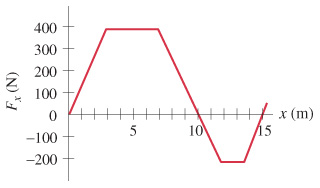# Problem: The force on a particle, acting along the x axis, varies as shown in the figure .Determine the work done by this force to move the particle along the x axis: from x = 0.0 to x = 10.0m.Determine the work done by this force to move the particle along the x axis: from x = 0.0 to x = 15.0m.

###### FREE Expert Solution
89% (188 ratings)
###### Problem Details

The force on a particle, acting along the x axis, varies as shown in the figure.

Determine the work done by this force to move the particle along the x axis: from x = 0.0 to x = 10.0m.

Determine the work done by this force to move the particle along the x axis: from x = 0.0 to x = 15.0m.

Frequently Asked Questions

What scientific concept do you need to know in order to solve this problem?

Our tutors have indicated that to solve this problem you will need to apply the Work By Variable Forces (Springs) concept. You can view video lessons to learn Work By Variable Forces (Springs). Or if you need more Work By Variable Forces (Springs) practice, you can also practice Work By Variable Forces (Springs) practice problems.